# ISEE Middle Level Math : Data Analysis

## Example Questions

### Example Question #41 : Data Analysis And Probability

Find the range for this set of numbers: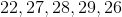Possible Answers:Correct answer:Explanation:

First, order the numbers from least to greatest: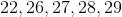Then, subtract the smallest number from the largest number: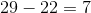Answer:### Example Question #42 : Data Analysis And Probability

Find the range in this set: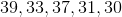Possible Answers:Correct answer:Explanation:

First order the number from least to greatest: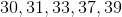Then, subtract the smallest from the largest number: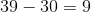Answer: The range is.

### Example Question #43 : Data Analysis And Probability

Find the range for this set of numbers: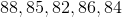Possible Answers:Correct answer:Explanation:

First, order the numbers from least to greatest: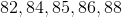Then, subtract the smallest number from the largest number: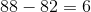Answer:### Example Question #44 : Data Analysis And Probability

Find the range in this set of numbers: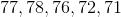Possible Answers:Correct answer:Explanation:

First, order the numbers from least to greatest: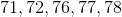Then, subtract the smallest number from the largest number: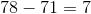Answer:### Example Question #45 : Data Analysis And Probability

Find the range for this set of numbers: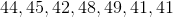Possible Answers:Correct answer:Explanation:

First, order the numbers from least to greatest: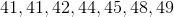Then, subtract the smallest number from the largest: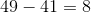Answer:### Example Question #46 : Data Analysis And Probability

Find the range for this set of numbers: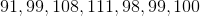Possible Answers: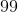Correct answer:Explanation:

First, order the numbers from least to greatest: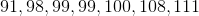Then, subtract the smallest number from the largest number: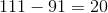Answer:### Example Question #47 : Data Analysis And Probability

Find the range in this set of numbers: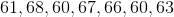Possible Answers:Correct answer:Explanation:

First order the numbers from least to greatest: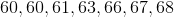Then, subtract the smallest number from the largest number: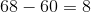Answer:### Example Question #48 : Data Analysis And Probability

Find the range for this set of numbers: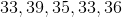Possible Answers:Correct answer:Explanation:

First, order the numbers from least to greatest: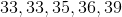Then, subtract the smallest number from the largest number: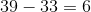Answer:### Example Question #49 : Data Analysis And Probability

Find the range in this set of number: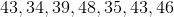Possible Answers:Correct answer:Explanation:

First order the numbers from least to greatest: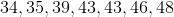Then, subtract the smallest number from the largest number: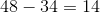Answer:### Example Question #43 : How To Find Range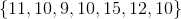Find the range of the data set above.

Possible Answers:Correct answer:Explanation:

To find the range, you must subtract the lowest term from the greatest term in the data set. The greatest term is 15. The lowest term is 9. Therefore, to find the range, you calculate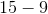. The answer is 6.

### All ISEE Middle Level Math Resources